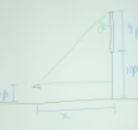# A rectangular billboard 9 feet in height stands in a field so that its bottom is 10 feet above...

## Question:

A rectangular billboard 9 feet in height stands in a field so that its bottom is 10 feet above the ground. A nearsighted cow with eye level at 4 feet above the ground stands {eq}x {/eq} feet from the billboard.

a. Express {eq}\theta(x) {/eq}, the vertical angle subtended by the billboard at her eye, in terms of {eq}x {/eq}.

b. Then find the distance the cow must stand from the billboard to maximize {eq}\theta(x) {/eq}.

## Vertical angle and angle of elevation:

Vertical angle is the angle subtended by the line of sight with respect to a vertical line at that point.

We can calculate this angle by the help of trigonometric identities that we have

For example {eq}\sin\theta = \frac{perpendicular}{hypotenuse} {/eq}

{eq}\cos\theta = \frac{base }{hypotenuse} {/eq}

and

{eq}\tan\theta = \frac{perpendicular}{base} {/eq}

{eq}\csc\theta = \frac{1}{\sin\theta} {/eq}

{eq}\sec\theta = \frac{1}{\cos\theta} {/eq}

and

{eq}\cot\theta = \frac{1}{\tan\theta} {/eq}

Become a Study.com member to unlock this answer! Create your account

As per the question, our figure should likea) Now the vertical angle {eq}\theta {/eq} can be calculated by finding the Tan of that angle.

We...